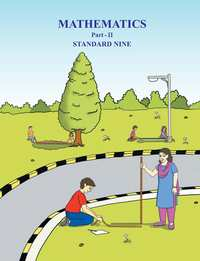Share

# Balbharati solutions for Class 9 chapter 4 - Constructions of Triangles [Latest edition]

#### Chapters## Chapter 4: Constructions of Triangles

Practice Set 4.1Practice Set 4.2Practice Set 4.3Problem Set 4

#### Balbharati solutions for Class 9 Chapter 4 Exercise Practice Set 4.1 [Page 53]

Practice Set 4.1 | Q 1 | Page 53

Construct Δ PQR, in which QR = 4.2cm m∠ Q = 40 ° and PQ + PR = 8.5cm

Practice Set 4.1 | Q 2 | Page 53

Construct  Δ XYZ, in which YZ = 6 cm, XY + XZ = 9 cm.
∠ XYZ = 50°

Practice Set 4.1 | Q 3 | Page 53

Construct  Δ ABC, in which BC = 6.2 cm,  ∠ ACB= 50° , AB + AC  = 9.8 cm

Practice Set 4.1 | Q 4 | Page 53

Construct  Δ ABC, in which BC =5.2 cm,  ∠ ACB = 45 ° and perimeter  of  Δ ABC  is 10 cm.

#### Balbharati solutions for Class 9 Chapter 4 Exercise Practice Set 4.2 [Page 54]

Practice Set 4.2 | Q 1 | Page 54

Construct Δ XYZ, such that  YZ = 7.4 cm, ∠XYZ = 45°  and  XY - XZ = 2.7 cm.

Practice Set 4.2 | Q 2 | Page 54

Construct Δ PQR, such that  QR = 7.4 cm, ∠PQR= 60 ° and  PQ - PR = 2.5 cm.

Practice Set 4.2 | Q 3 | Page 54

Construct Δ ABC, such that BC = 6 cm, ∠ABC = 100°   and  AC - AB = 2.5cm.

#### Balbharati solutions for Class 9 Chapter 4 Exercise Practice Set 4.3 [Page 56]

Practice Set 4.3 | Q 1 | Page 56

Construct Δ PQR,  in which ∠Q = 70°,  ∠R = 80° and PQ + QR + PR = 9.5 cm.

Practice Set 4.3 | Q 2 | Page 56

Construct Δ XYZ, in which ∠Y = 58°, ∠X = 46° and  perimeter of triangle is 10.5 cm.

Practice Set 4.3 | Q 3 | Page 56

Construct Δ LMN, in which ∠M = 60°, ∠N = 80°  and  LM + MN + NL = 11 cm.

#### Balbharati solutions for Class 9 Chapter 4 Exercise Problem Set 4 [Page 56]

Problem Set 4 | Q 1 | Page 56

Construct Δ XYZ, such that  XY + YZ  =10.3 cm,  YZ = 4.9 cm, ∠XYZ = 45° .

Problem Set 4 | Q 2 | Page 56

Construct Δ ABC, in which ∠B = 70°, ∠C = 60° , AB + BC + AC = 11.2 cm.

Problem Set 4 | Q 3 | Page 56

The perimeter of a triangle is 14.4 cm and the ratio of lengths of its side is 2 : 3 : 4. Construct the triangle.

Problem Set 4 | Q 4 | Page 56

Construct Δ PQR, in which PQ - PR = 2.4 cm, QR = 6.4 cm and ∠PQR = 55° .

## Chapter 4: Constructions of Triangles

Practice Set 4.1Practice Set 4.2Practice Set 4.3Problem Set 4## Balbharati solutions for Class 9 Geometry chapter 4 - Constructions of Triangles

Balbharati solutions for Class 9 chapter 4 (Constructions of Triangles) include all questions with solution and detail explanation. This will clear students doubts about any question and improve application skills while preparing for board exams. The detailed, step-by-step solutions will help you understand the concepts better and clear your confusions, if any. Shaalaa.com has the Maharashtra State Board Balbharati Class 9 Mathematics 2 Geometry solutions in a manner that help students grasp basic concepts better and faster.

Further, we at Shaalaa.com provide such solutions so that students can prepare for written exams. Balbharati textbook solutions can be a core help for self-study and acts as a perfect self-help guidance for students.

Concepts covered in Class 9 Geometry chapter 4 Constructions of Triangles are Perpendicular bisector Theorem, Construction of Triangles.

Using Balbharati Class 9 solutions Constructions of Triangles exercise by students are an easy way to prepare for the exams, as they involve solutions arranged chapter-wise also page wise. The questions involved in Balbharati Solutions are important questions that can be asked in the final exam. Maximum students of Maharashtra State Board Class 9 prefer Balbharati Textbook Solutions to score more in exam.

Get the free view of chapter 4 Constructions of Triangles Class 9 extra questions for and can use Shaalaa.com to keep it handy for your exam preparation

S